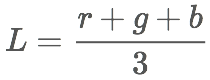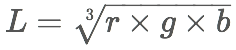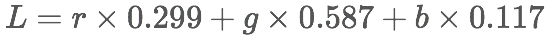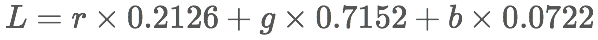# A Closer Look at Color Lightness

As much as we strive to control every detail of what we build, we are sometimes left with little choice but to leave some design decisions to algorithms. The challenges associated with these decisions become a lot more significant when human perception comes into play. One common example: programmatically choosing a foreground color based on a given background color.

It may sound trivial at first, but having a closer look reveals that there is a bit more to it under the surface. A quick Google search will give some hints, but it will probably end up in more confusion, not least due to the inaccurate use of terms related to the topic.

So, let’s get this straight.

## A bit of terminology

Before crunching any numbers, let’s define some key concepts relating to colors in the context of screens:

• Brightness: In a broad sense, brightness is the attribute of an object emitting or reflecting light. More specifically, it’s the arithmetic mean of the three components in the RGB—red, green, blue— color model or the third component in the HSB—hue, saturation, brightness—model.
• Radiance: The total amount of light that goes through a particular area.
• Weighted: We do not perceive all wavelengths the same; for instance, we tend to perceived green to be lighter than blue or red. A weighted attribute is an attribute that takes that into consideration by giving more weight to green, and less to red and blue.
• Gamma correction: The process of coding and decoding luminance with the aim of optimizing the output for human vision.
• Luma: Used primarily in video, luma is the weighted sum of gamma-corrected RGB values.
• Lightness: A subjective measure of brightness, relative to the brightness of a white point.
• Normalization: The process of expressing lightness as a ratio corresponding to the absolute lightness value of the color to that of pure white.

In short, brightness is an absolute measure of light emitted or reflected from an object, while lightness is a subjective measure of perceived light.

## How to Determine Lightness

There are several ways to approximate the lightness of a color, with varying degrees of precision. Some are weighted—they take into consideration how we perceive colors—and some are not. For simplicity’s sake, we will refer to the value of lightness as `L` throughout this section. Pure red (`255,0,0`) will be used as an example.

### Non-weighted Methods

These formulae do not take into consideration the perceived lightness of the primary and secondary colors. In other words you’d find out similar results for hues that are primarily red and ones that are primarily green.

#### Using HSB Brightness

The brightness component of the HSB model corresponds to the value of the largest RGB component:Ex: Pure red would have an HSB value of `255` (`1.0` normalized) since both other components are null.

#### Using HSL Lightness

The lightness component of the HSL—hue, saturation, lightness—model corresponds to the arithmetic mean of the largest and smallest RGB components:Ex: Pure red would have an HSL lightness of `127.5` (`0.5` normalized).

#### Using Intensity

Intensity is the average of the three components in the RGB space. It could be calculated using an arithmetic mean…or a geometric one:Ex: Pure red would have an arithmetic intensity of `85` (`0.33` normalized), and a geometric intensity of `0`.

#### Using Euclidean Distance in 3D RGB space

Considering a three-dimensional, cube-shaped RGB space, lightness would correspond to the Euclidean distance between the color point and the space origin (black):Ex: Pure red would have a euclidean lightness of `255` (`0.57` normalized).

### Weighted Methods

Weighted formulae take into consideration the perceived lightness of the three primaries, by giving each a coefficient corresponding to how light or dark the human eye perceives it. In video, weighted lightness is commonly referred to as luma.

#### W3C Method (Working Draft)Ex: Pure red would have a W3C lightness of `76` (`0.299` normalized).

#### sRGB Luma (Rec. 709)Ex: Pure red would have a lightness of `54.2` (`0.21` normalized).

#### Using Weighted Euclidean Distance in 3D RGB Space

This method is not official, but it has been reported that it produces better results than the previous two:Ex: Pure red would have a lightness of `125.1` (`0.49` normalized).

## Tests

In order to determine which of these methods is more reliable, we need to test them with different colors and lightness thresholds, then aggregate the results.

• Each color will have a subjective, expected outcome (dark or light).
• If the test result matches the expected outcome, it will be assigned a value of `1`. Otherwise it is `0`.
• The higher the score of a method/threshold combination, the more reliable it is.

The tests will be manually run using this online tool made specifically for the purpose.

## Results & Conclusion

• HSB brightness with a `.5` threshold yielded the least accurate results.
• Weighted Euclidean distance (`.7`) and sRGB luma (`.6`) performed best within this test.